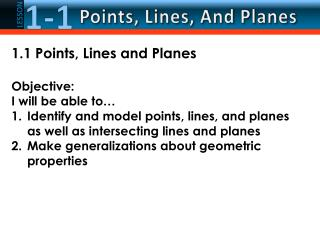DownloadDownload Presentation1.1 Points, Lines and Planes Objective: I will be able to…

# 1.1 Points, Lines and Planes Objective: I will be able to…

Download Presentation## 1.1 Points, Lines and Planes Objective: I will be able to…

- - - - - - - - - - - - - - - - - - - - - - - - - - - E N D - - - - - - - - - - - - - - - - - - - - - - - - - - -
##### Presentation Transcript

1. 1.1 Points, Lines and Planes Objective: I will be able to… • Identify and model points, lines, and planesas well as intersecting lines and planes • Make generalizations about geometric properties

2. Undefined Terms Point: a location with no dimensions. Usually represented by a dot and a capital letter. A pt A Line: made of points that extend in one dimension – no width or depth, but infinite length. 2 points determine a line. l A B line l

3. Plane: made of points that extend infinitely in two directions, but has no height. A flat surface with no thickness. Three noncollinearpoints determine and name a plane. A capital script letter can also name a plane. A M B D C

4. Defined Term: items defined by means of undefined terms or previously defined terms. Collinear: points or other objects that all lie on one line. C D D A B

5. Defined Term: items defined by means of undefined terms or previously defined terms. Coplanar: points or other objects that all lie on one plane. M A B C D

6. Answer: The line can be named as line a. There are three points on the line. Any two of the points can be used to name the line. EXAMPLE 1 A. Use the figure to name a line containing point K. Example 1

7. Answer: The plane can be named as plane B. You can also use the letters of any three noncollinearpoints to name the plane.plane JKM plane KLM plane JLM EXAMPLE 1 B. Use the figure to name a plane containing point L. There are 15 different three-letter names for this plane (any order) Example 1

8. EXAMPLE 1 A. Use the figure to name a line containing the point X. Line c B. Use the figure to name a plane containing point Z. Plane P Example 1a

9. 1-1 Points, Lines, And Planes LESSON Real World EXAMPLE 2 A. Name the geometric shape modeled by a 10  12 patio. Answer:The patio models a plane. Example 2

10. Real World EXAMPLE 2 A. Name the geometric shape modeled by a colored dot on a map used to mark the location of a city. Point Example 2a

11. Real World EXAMPLE 2 B. Name the geometric shape modeled by the ceiling of your classroom. Plane Example 2b

12. Name a line that contains point A. Try 2-5 on your own… 2. What is another name for line ℓ? 3. Name a point not on .

13. EXAMPLE 3 Draw a surface to represent plane R and label it. Example 3

14. EXAMPLE 3 Draw a line anywhere on the plane. Example 3

15. EXAMPLE 3 Draw dots on the line for point A and B. Label the points. Example 3

16. EXAMPLE 3 Example 3

17. EXAMPLE 3 Draw dots on this line for point D and E. Label the points. Example 3

18. EXAMPLE 3 Label the intersection point of the two lines as P. Example 3

19. EXAMPLE 3 Answer: Example 3

20. A. B.C.D. EXAMPLE 3 A. Choose the best diagram for the given relationship. Plane D contains line a, line m, and line t, with all three lines intersecting at point Z. Also, point F is on plane D and is not collinear with any of the three given lines. Example 3a

21. EXAMPLE 4 A. How many planes appear in this figure? Answer: There are two planes: plane S and plane ABC. Example 4

22. EXAMPLE 4 B. Name three points that are collinear. Answer: Points A, B, and D are collinear. Example 4

23. Try on your own! Refer to the figure. How many planes are shown in the figure? How many of the planes contain points F and E? Name four points that are coplanar. Are points A, B, and C coplanar? Explain.

24. 1. Name the intersection of plane N and line . 2. Name the intersection of and . 3. Does intersect ? Explain.

25. Example 6 What is this?

26. Example 6 What is this?

27. Example 6 ABC What is this?

28. Undefined term: a term that is only explained using examples and descriptions Point: a location with no dimensions; it has no shape or size Line: made up of points and has no thickness or width (1 dimension); must have 2 points for a line Plane: a flat surface made up of points that extends infinitely in all directions (2 dimensions); must have 3 non-collinear points for a plane

29. Collinear: points that lie on the same line Coplanar: points that lie on the same plane Intersection: the set of points they have in common What do 2 intersecting lines have in common? What do 2 intersecting planes have in common? What do an intersecting line and a plane have in common? Defined term: explained using undefined terms and/or other defined terms